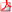# Publications

### Articles publiés

1. Buyukboduk K, Lei A, Venkat G, “Iwasawa theory for symmetric square of non-p-ordinary eigenforms”, Documenta Mathematica, 26, 2021, 1–632. Lei A, Sujatha R, “On Selmer groups in the supersingular reduction case”, Tokyo Journal of Mathematics, 43 (2), 2020, 455–4793. Bellemare H, Lei A, “Explicit uniformizers for certain totally ramified extensions of the field of p-adic numbers”, Abhandlungen aus dem Mathematischen Seminar der Universität Hamburg, 90 (1), 2020, 73–834. Lei A, Poulin A, “On certain probabilistic properties of polynomials over the ring of p-adic integers”, American Mathematical Monthly, 127 (6), 2020, 519–5295. Delbourgo D, Lei A, “Heegner cycles and congruences between anticyclotomic p-adic L-functions over CM-extensions”, The New York Journal of Mathematics, 26, 2020, 496–5256. Lei A, Sprung F, “Ranks of elliptic curves over Z_p^2-extensions”, Israel Journal of Mathematics, 236 (1), 2020, 183–2067. Buyukboduk K, Lei A, “Functional Equation for p-adic Rankin-Selberg L-functions”, Annales mathématiques du Québec, 44 (1), 2020, 9–258. Lei A, Ponsinet G, “On the Mordell-Weil ranks of supersingular abelian varieties in cyclotomic extensions”, Proceedings of the American Mathematical Society Series B, 7, 2020, 1-169. Buyukboduk K, Lei A, “Rank–two Euler systems for symmetric squares”, Transactions of the American Mathematical Society,  372 (12), 2019, 8605-861910. Hatley J, Lei A, “Comparing anticyclotomic Selmer groups of positive coranks for congruent modular forms”, Mathematical Research Letters, 26 (4), 2019, 1115-114411. Lei A, Palvannan B, “Codimension two cycles in Iwasawa theory and elliptic curves with supersingular reduction”, Forum of Mathematics, Sigma, 7, 2019, e2512. Hatley J, Lei A, “Arithmetic properties of signed Selmer groups at non-ordinary primes”, Annales de l’Institut Fourier, 69 (3) 2019, 1259-129413. Buyukboduk K, Lei A, Loeffler D, Venkat G, “Iwasawa theory for Rankin–Selberg products of p-non-ordinary eigenforms”, Algebra & Number Theory, 13(4), 2019, 901-94114. Lei A, Loeffler D, Zerbes S, “Euler systems for Hilbert modular surfaces”, Forum of Mathematics, Sigma, 6, 2018 , e2315. Delbourgo D, Lei A, “Congruences modulo p between rho-twisted Hasse-Weil L-values”, Transactions of the American Mathematical Society, 370 (11), 2018, 8047-808016. Buyukboduk K, Lei A, “Anticyclotomic p-ordinary Iwasawa theory of elliptic modular forms”, Forum Mathematicum, 30 (4), 2018, 887–91317. Lei A, “Bounds on the Tamagawa numbers of a crystalline representation over towers of cyclotomic extensions”, Tohoku Mathematical Journal, 69 (4), 2017, 497-52418. Dion, C, Lei, A “Plus and minus logarithms and Amice transform”, Comptes Rendus Mathématiques, 355 (9), 2017, 942-94819. Lei A, “Estimating class numbers over metabelian extensions”, Acta Arithmetica, 180 (4), 2017, 347-36420. Lei A, Loeffler D, Zerbes S, “On the asymptotic growth of Bloch-Kato-Shafarevich-Tate groups of modular forms over cyclotomic extensions”, Canadian Journal of Mathematics, 69 (4), 2017, 826-85021. Buyukboduk K, Lei A, “Integral Iwasawa Theory of Galois Representations for non-ordinary primes”, Mathematische Zeitschrift, 286 (1), 2017, 361-39822. Lei A, Ponsinet G, “Functional equations for multi-signed Selmer groups”, Annales mathématiques du Québec, 41 (1), 2017, 155-16723. Delbourgo D, Lei A, “Estimating the growth in Mordell-Weil ranks and Shafarevich-Tate groups over Lie extensions”, The Ramanujan Journal, 43 (1), 2017, 29-6824. Ayotte D, Lei A, Rondy-Turcotte J-C, “On the parity of supersingular Weil polynomials”, Archiv der Mathematik, 106 (4), 2016, 345-35325. Delbourgo D, Lei A, “Non-commutative Iwasawa theory for elliptic curves with multiplicative reduction”, Mathematical Proceedings of the Cambridge Philosophical Society, 160 (1), 2016, 11-3826. Buyukboduk K, Lei A, “Coleman-adapted Rubin-Stark Kolyvagin systems and supersingular Iwasawa theory of CM abelian varieties”, Proceedings of the London Mathematical Society, 111 (6), 2015, 1338-137827. Delbourgo D, Lei A, “Transition formulae for ranks of abelian varieties”, Rocky Mountain Journal of Mathematics, 45 (6), 2015, 1807-183828. Lei A, Loeffler D, Zerbes S, “Euler systems for modular forms over imaginary quadratic fields”, Compositio Mathematica, 151 (9), 2015, 1585-162529. Harron R, Lei A, “Iwasawa theory for symmetric powers of CM modular forms at non-ordinary primes”, Journal de théorie des nombres de Bordeaux, 26(3), 2014, 673-70730. Lei A, “Factorisation of two-variable p-adic L-functions”, Canadian Mathematical Bulletin, 57(4), 2014, 845-85231. Lei A, Loeffler D, Zerbes S, “Euler systems for Rankin-Selberg convolutions of modular forms”, Annals of Mathematics, 180(2), 2014, 653-77132. Lei A, Loeffler D, Zerbes S, “Critical Slope p-adic L-functions of CM Modular Forms”, Israel Journal of Mathematics, 198(1), 2013, 261-28233. Lei A, “Non-commutative p-adic L-functions for Supersingular Primes”, International Journal of Number Theory, 8(8), 2012, 1813-183034. Lei A, Zerbes S, “Signed Selmer Groups over p-adic Lie Extensions”, Journal de théorie des nombres de Bordeaux, 24(2), 2012, 377-40335. Lei A, “Iwasawa Theory for the Symmetric Square of a CM Modular Forms at Inert Primes”, Glasgow Mathematical Journal, 54(2), 2012, 241-25936. Lei A, Loeffler D, Zerbes S, “Coleman Maps and p-adic Regulator”, Algebra and Number Theory, 5(8), 2011, 1095-113137. Lei A, “Iwasawa Theory for Modular Forms at Supersingular Primes”, Compositio Mathematica, 147(3), 2011, 803-83838. Lei A, Loeffler D, Zerbes S, “Wach Modules and Iwasawa Theory for Modular Forms”, Asian Journal of Mathematics, 14(4), 2010, 475-52839. Lei A, “Coleman Maps for Modular Forms at Supersingular Primes over Lubin-Tate Extensions”, Journal of Number Theory, 130(10), 2010, 2293-2307